# Previous Year Questions - Structure of Atom, Class 11 (IIT - JEE Advanced) Notes | Study Chemistry for JEE Advanced - JEE

## JEE: Previous Year Questions - Structure of Atom, Class 11 (IIT - JEE Advanced) Notes | Study Chemistry for JEE Advanced - JEE

The document Previous Year Questions - Structure of Atom, Class 11 (IIT - JEE Advanced) Notes | Study Chemistry for JEE Advanced - JEE is a part of the JEE Course Chemistry for JEE Advanced.
All you need of JEE at this link: JEE

Previous Year Questions - Structure of Atom, Class 11 (IIT - JEE Advanced)

Q 1. With what velocity should an α-particle travel towards the nucleus of a Cu atom so as to arrive at a distance 10-13 m.                 [JEE 1997]

Ans. 6.3×108 m/s

Q 2. A compound of Vanadium has magnetic moment of 1.73 BM work out electronic configuration of Vanadium Ion in the compound.      [JEE 1997]

Ans. 23V+2 : [Ar] 3d1

Q 3. Calculate the energy required to excite one litre of hydrogen gas at 1 atm and 298 K to the first excited state of atomic hydrogen. The energy for the dissociation of H - H is 436 kJ mol-1

Ans. 98.2 kJ

Q4. The Schrodinger wave equation for hydrogen atom is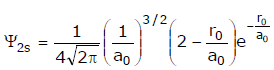Where a0 is Bohr's radius. If the radial node is 2s be at r0, then find r0 in term of a0.            [JEE 2004]

Ans. 2a0

Q 5. A ball of mass 100 g is moving with 100 m/s. Find its wavelength                          [JEE 2004]

Ans. 6.6 × 10-35 s-1

Q 6. Find the velocity (m/s) of electron in first Bohr's orbit of radius a0. Also find the de Broglie's wavelength (in m). Find the orbital angular momentum of 2p orbital of hydrogen atom in units of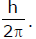[JEE 2004]

Ans. 3.34 × 10-10 m

Q 7. The maximum number of electrons that can have principal quantum number, n = 3, and spin quantum number, ms = – 1/2, is:            [JEE 2011]

Ans. 0009

Q 8. The work function (φ) of some metals is listed below. The number of metals which will show photoelectric effect when light of 300 nm wavelength falls on the metal is                [JEE 2011]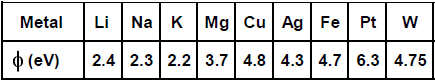Ans. 0004

Q 9. The work functions of Silver and Sodium are 4.6 and 2.3 eV, respectively. The ratio of the slope of the stopping potential versus frequency plot for Silver to that of Sodium is                [JEE 2013]

Ans. 1

Q 10. The atomic masses of He and Ne are 4 and 20 a.m.u. respectively. The value of the de Broglie wavelength of He gas at -73 ºC is "M" times that of the de Broglie wavelength of Ne at 727 ºC. M is [JEE 2013]

Ans. 0005

------------------------------------------------------------------------------------------------------------------------------------------------------

Previous Year Questions - Organic Chemistry, Class 11 (IIT - JEE Advanced)

Q 1. Out of anhydrous AlCl3 and hydrous AlCl3 which is more soluble in diethyleither ? Explain with reason.                     [JEE-2003]

Sol. Anhydroush AlCl3 is more soluble in diethyl ether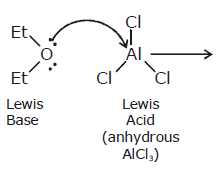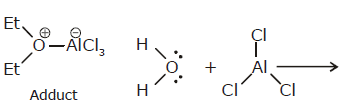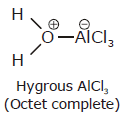Q 2. Match Ka values with suitable acid:              [JEE-2003]

Ka
(i) 3.3 × 10-5
(ii) 4.2 × 10-5
(iii) 6.3 × 10-5
(iv) 6.4 × 10-5
(v) 30.6 × 10-5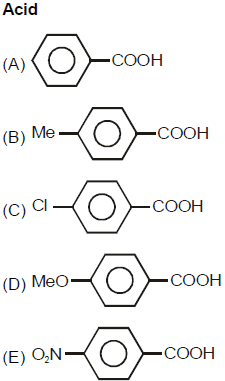Ans. (i) d

(ii) b

(iii) a

(iv) c

(v) e

Q 3. Give resonating structures of following compound: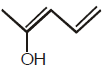[JEE-2003]

Ans.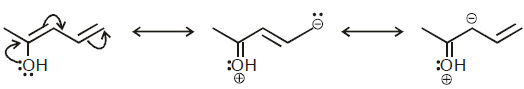Q 4. Which of the following is more acidic and why ?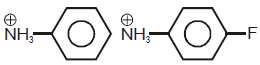[JEE-2004]

Ans.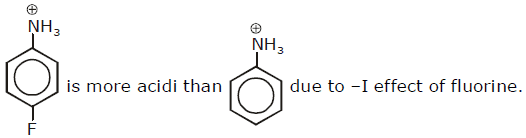Q 5. Predict whether the following molecules are iso structural or not. Justify your answer.  [JEE-2005]
(i) NMe(3

(ii) N(SiMe3)3

Ans.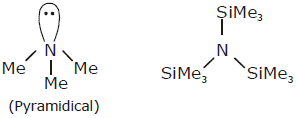Given componds are not isostrucutral
Delocalised of l.p. of nitrogen in vacant d-orbital of silicon makes compound planar.

Q 6. The total number of basic group in the following form of lysine is               [JEE-2010]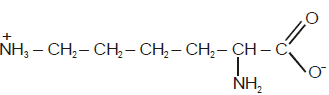Ans. 2

Q 7. The total number of contrubting structure showing hyperconjugation (involving C-H bonds) for the following carbocation is                                      [JEE-2011]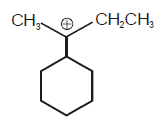Ans. 6

------------------------------------------------------------------------------------------------------------------------------------------------------

Previous Year Questions - States of Matter - Gases and Liquids, Class 11 (IIT - JEE Advanced)

Q. 1 The density of the vapour of a substance at 1 atm pressure and 500 K is 0.36 Kg m-3. The vapour effuses through a small hole at a rate of 1.33 times faster than oxygen under the same condition.
[JEE 2002]

(a) Determine
(i) mol. wt.;
(ii) molar volume;
(iii) compression factor (z) of the vapour and
(iv) which forces among the gas molecules are dominating, the attractive or the repulsive

(b) If the vapour behaves ideally at 1000K , determine the average translational K.E. of a molecule.

Ans.

(a)

(i) 18.1 g/mol ,

(ii) 50.25 L mol–1 ,

(iii) 1.224 ,

(iv) repulsive,

(b) 2.07 × 10–20 J

Q 2. The average velocity of gas molecules is 400 m/sec. Calculate its (rms) velocity at the same temperature.                                                                       [JEE 2003]

Ans. 434.17 m/sec

Q 3. CV value of He is always 3R/2 but CV value of H2 is 3R/2 at low temperature and 5R/2 at moderate temperature and more than 5R/2 at higher temperature explain in two to three lines. [JEE 2003]

Ans. Since H2 is diatomic and He is monoatomic degree of freedom for mono is 3 and only translational
but for diatomic, vibrational and rotational are also to be considered

Q 4. For a real gas obeying van der Waal's equation a graph is plotted between PVm (y-axis) and P(x-axis) where Vm is molar volume. Find y-intercept of the graph.                           [JEE 2004]

Ans. RT

Q 5. The atomic masses of He and Ne are 4 and 20 a.m.u, respectively. The value of the de Broglie wavelength of He gas at -73°C is “M” times that of the de Broglie wavelength of Ne 727°C. M is:

Ans. 5

------------------------------------------------------------------------------------------------------------------------------------------------------

Previous Year Questions - Thermochemistry, Class 11 (IIT - JEE Advanced)

Q 1. Estimate the average S - F bond energy in SF6. The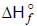values of SF6(g), S(g), and F(g) are -1100, 275 and 80 kJ/mol respectively.                               [JEE 1999]

Ans. 309.6 kJ/mol

Q 2. Diborane is potential rocket fuel which undergoes combustion according to the reaction,
B2H6(g) + 3O2(g) → B2O3(s) + 3H2O (g)
From the following data, calculate the enthalpy change for the combustion of diborane :

2B(s) +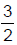O2(g) → B2O3(s) ;                  ΔH = –1273 kJ
H2(g) +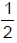O2(g) → H2O(l) ;                   ΔH = –286 kJ
H2O (l) → H2O(g) ;                                 ΔH = 44 kJ
2B(s) + 3H2(g) → B2H6(g) ;                    ΔH = 36 kJ                      [JEE 2000]

Ans. -2035 kJ mol-1

Q 3. In a constant volume calorimeter, 3.5 g of a gas with molecular weight 28 was burnt in excess oxygen at 298.0 K. The temperature of the calorimeter was found to increase from 298.0 K to 298.43 K due to the combustion process. Given that the heat capacity of the calorimeter is 2.5 kJ K-1, the numerical value for the enthalpy of combustion of the gas in KJ mol–1 is                      [JEE 2009]

Ans. 9

------------------------------------------------------------------------------------------------------------------------------------------------------

Previous Year Questions - Isomerism, Class 11 (IIT - JEE Advanced)

Q 1

(i)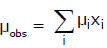whre µi is the dipole moment of a stable conformer of the molcule, Z - CH2 - CH2 - Z and xi is the mole fraction of the stable conformer.

Given : µobs = 1.0 D and x (Anti) = 0.82

Draw all the stable conformers of Z - CH2 - CH2 - Z and calculate the value of µ(Gauche).

(ii) Draw the stable conformer of Y - CHD - CHD - Y (meso form), when Y = CH3 (rotation about C2 - C3) and Y = OH (rotation about C1 - C2) in Newmann projection.                     [JEE 2005]

Ans.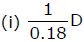(ii) Anti form when Y = CH3 & Gauche when Y = -OH

Q 2. The total number of cyclic structural as well as stereo isomers possible for compound with the molecular formular C5H10 is                   [JEE 2009]

Ans. 7

Q 3. The total number of cyclic isomers possible for a hydrocarbon with the molecular formula C4H6 is                                 [JEE 2010]

Ans. 5

------------------------------------------------------------------------------------------------------------------------------------------------------

Previous Year Questions - Chemical Equilibrium, Class 11 (IIT - JEE Advanced)

Q 1. The degree of dissociation is 0.4 at 400K & 1.0 atm for the gasoeus reaction PCl5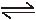PCl3+ Cl2(g). Assuming ideal behaviour of all gases. Calculate the density of equilibrium mixture at 400K & 1.0 atm pressure. [JEE 1999]

Ans. 4.54 g dm–3

Q 2. When 3.06 g of solid NH4HS is introduced into a two litre evacuated flask at 27°C, 30% of the solid decomposes into gaseous ammonia and hydrogen sulphide.      [JEE 2000]

(i) Calculate KC & KP for the reaction at 27°C.

(ii) What would happen to the equilibrium when more solid NH4HS is introduced into the flask ?

Ans. (i) Kc = 8.1 × 10–5 mol2 L2 ; Kp = 4.91 × 10–2 atm2

(ii) No effect ;

------------------------------------------------------------------------------------------------------------------------------------------------------

Previous Year Questions - Ionic Equilibrium, Class 11 (IIT - JEE Advanced)

Q 1. What will be the resultant pH when 200 ml aqueous solution of HCl (pH = 2.0) is mixed with 300 ml of an aqueous solution of NaOH (pH=12.0)?                [JEE 1998]

Ans. pH=11.3010

Q 2. The solubility of Pb(OH)2 in water is 6.7 × 10-6 M. Calculate the solubility of Pb(OH)2 in a buffer solution of pH= 8.                        [JEE '1999]

Ans. s = 1.203×10-3 M

Q 3. 500 ml of 0.2 M aqueous solution of acetic acid is mixed with 500 mL of 0.2 M HCl at 25°C

(a) Calculate the degree of dissociation of acetic acid in the resulting solution and pH of the solution.

(b) If 6 g of NaOH is added to the above solution, determine final pH. Assume there is no change in volume on mixing. Ka of acetic acid is 1.75 × 10-5 M.                    [JEE 2002]

Ans. (a) 0.0175%

(b) 4.757

Q 4. Will the pH of water be same at 4°C and 25°C ? Explain.                      [JEE 2003]

Ans. No it will be > 7

Q 5. 0.1 M of HA is titrated with 0.1 M NaOH, calculate the pH at end point. Given Ka(HA) = 5 × 10-6 and a << 1.                       [JEE 2003]

Ans. pH = 9

Q 6. The dissociation constant of a substituted benzoic acid at 25ºC is 1.0 × 10-4. The pH of a 0.01 M solution of its sodium salt is :               [JEE 2009]

Ans. pH = 8

Q 7. The total number of diprotic acids among the following is                   [IIT-2010]

H3PO4 H2SO4 H3PO3 H2CO3 H2S2O7

H3BO3 H3PO2 H2CrO4 H2SO3

Ans. 6

Q 8. In 1 L saturated solution AgCl [Ksp(AgCl) = 1.6 × 10-10], 0.1 mol of CuCl [Ksp(CuCl) = 1.0 × 10-6] is added. The resultant concentration of Ag+ in the solution is 1.6 × 10-x. The value of "x" is

[JEE 2011]

Ans. 7

------------------------------------------------------------------------------------------------------------------------------------------------------

Previous Year Questions - Stoichiometry, Class 11 (IIT - JEE Advanced)

Q 1. How many moles of e_ weigh one Kg

Ans .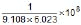Q 2. Calculate the molarity of pure water using its density to be 1000 kg m-3.               [JEE' 2003]

Ans. 55.5 mol L-1

Q 3. One gm of charcoal absorbs 100 ml 0.5 M CH3COOH to form a monolayer, and there by the molarity of CH3COOH reduces to 0.49. Calculate the surface area of the charcoal adsorbed by each molecule of acetic acid. Surface area of charcoal = 3.01 × 102 m2/gm.

Ans. 5 × 10-19 m2

Q 4. Calculate the amount of Calcium oxide required when it reacts with 852 gm of P4O10. [JEE 2005]

Ans. 1008 gm

Q 5. 20% surface sites have adsorbed N2. On heating N2 gas evolved from sites and were collected at 0.001 atm and 298 K in a container or volume is 2.46 cm3. Density of surface sites is 6.023 × 1014/cm2 and surface area is 1000 cm2, find out the no. of surface sites occupied per molecule of N2.

Ans. 0002

Q 6. Dissolving 120 g of urea (mol. wt. 60) in 1000 g of water gave a solution of density 1.15 g/mL. The molarity of the solution is:                                [JEE 2011]

Ans. 2.05 M

Q 7. Reaction of Br2 with Na2CO3 in aqueous solution gives sodium bromide and sodium bromate with evolution of CO2 gas. The number of sodium bromide molecules involved in the balanced chemical equation is:                                          [JEE 2011]

Ans. 0005

Q 8. A decapeptide (Mol. wt. 796) on complete hydrolysis gives glycine (Mol. Wt. 75), alanine and phenylalanine. Glycine contributes 47.0% to the total weight tto the hydrolysed products. The number of glycine units present in the decapeptideis : [JEE 2011]

Ans. 0006

The document Previous Year Questions - Structure of Atom, Class 11 (IIT - JEE Advanced) Notes | Study Chemistry for JEE Advanced - JEE is a part of the JEE Course Chemistry for JEE Advanced.
All you need of JEE at this link: JEEUse Code STAYHOME200 and get INR 200 additional OFF

### Up next12 videos|53 docs|118 tests

### Up nextTrack your progress, build streaks, highlight & save important lessons and more!

,

,

,

,

,

,

,

,

,

,

,

,

,

,

,

,

,

,

,

,

,

,

,

,

;